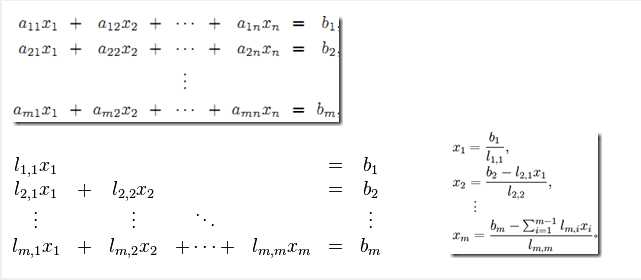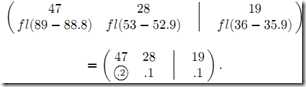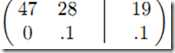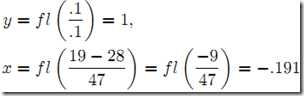# 1、线性方程组

1）构造增广矩阵，即系数矩阵A增加上常数向量b（A|b）

2）通过以交换行、某行乘以非负常数和两行相加这三种初等变化将原系统转化为更简单的三角形式（triangular form）

注：这里的初等变化可以通过系数矩阵A乘上初等矩阵E来实现

3）从而得到简化的三角方阵组，注意它更容易解

4）这时可以使用向后替换算法（Algorithm for Back Substitution）求解得

z=-4/-4=1,  y=4-2z=4-2=2,  x= (1-y-z)/2=(1-2-1)/2=-1

原线性方程组       ——>       高斯消元法     ——> 下三角或上三角形式的线性方程组           ——>  前向替换算法求解（对于上三角形式，采用后向替换算法）## 高斯－若尔当消元法（Gauss-Jordan Elimination）。此时，因为不能将第2行第1列位置变为0，所以不能将其三角化。从而，我们只能接受将这个位置值赋为0，而不管其实际浮点值。因此，3位浮点高斯消元的结果为，后向算法计算结果为`下面给出列主元消去的代码（所谓列主元消去法是在系数矩阵中按列选取元素绝对值得最大值作为主元素，然后交换所在行与主元素所在行的位置，再按顺序消去法进行消元。）`
``` 1 列选主元消元法
2 /*
3 *Gauss.cpp
4 *功能：列选主元消元法
5 */
6 #include<stdio.h>
7 #include"Gass.h"
8
9 //高斯消元法（列选主元）
10 void Gauss (double a[][MAXNUM],int n)
11 {
12     int i,j;
13     SelectColE(a,n);   //列选主元并消元成上三角
14     //回代求解
15     printf("上三角的结果\n");
16     printM(a,3);
17     for(i=n;i>=1;i--)
18     {
19         for(j=i+1;j<=n;j++)
20             a[i][n+1]-=a[i][j]*a[j][n+1];
21         a[i][n+1]/=a[i][i];
22     }
23     return ;
24 }
25 //选择列主元并进行消元
26 void SelectColE(double a[][MAXNUM],int n)
27 {
28     int i,j,k,maxRowE;
29     double temp; //用于记录消元时的因数
30     for(j=1;j<=n;j++)
31     {
32         maxRowE=j;
33         for(i=j;i<=n;i++)
34             if(fabs(a[i][j])>fabs(a[maxRowE][j]))
35                 maxRowE = i;
36         if(maxRowE!=j)
37             swapRow(a,j,maxRowE,n);   //与最大主元所在行交换
38         //消元
39         for(i=j+1;i<=n;i++)
40         {
41             temp =a[i][j]/a[j][j];
42             for(k=j;k<=n+1;k++)
43                 a[i][k]-=a[j][k]*temp;
44         }
45         printf("第%d列消元后：\n",j);
46         printM(a,3);
47     }
48     return;
49 }
50 void swapRow(double a[][MAXNUM],int m,int maxRowE,int n)
51 {
52     int k;
53     double temp;
54     for(k=m;k<=n+1;k++)
55     {
56         temp = a[m][k];
57         a[m][k] = a[maxRowE][k];
58         a[maxRowE][k] = temp;
59     }
60     return ;
61 }
62
63 //测试函数
64 int main()
65 {
66     double a[MAXNUM];
67
68     int i,n,j;
69
70     a = 0.001; a=2.000;a=3.000;a=1.000;
71     a = -1.000;a=3.712;a=4.623;a=2.000;
72     a = -2.000;a=1.070;a=5.643;a=3.000;
73     Gauss (a,3);
74     for(i=1;i<=3;i++)
75         printf("X%d=%f\n",i,a[i]);
76     return 0;
77 }
78 //输出矩阵
79 void printM(double a[][MAXNUM], int n)
80 {
81    int i,j;
82    for(i=1;i<=n;i++)
83     {
84         for(j=1;j<=n+1;j++)
85         {
86             printf("%f ,",a[i][j]);
87         }
88         printf("\n");
89     }
90 }```
`测试结果：`

# 2、逆矩阵(我们设方程组中方程的个数为equ，变元的个数为var，注意：一般情况下是n个方程，n个变元，但是有些题目就故意让方程数与变元数不同)

1. 把方程组转换成增广矩阵。

2. 利用初等行变换来把增广矩阵转换成行阶梯阵。

3. 转换为行阶梯阵，判断解的情况。

① 无解

② 唯一解

③ 无穷解。

这里单独介绍下这种解法：

```  1 #include <iostream>
2 #include <cmath>
3 #include <algorithm>
4
5 using namespace std;
6
7 const double eps = 1e-6;
8
9 bool is_zero( const double num )//用于判断有无逆矩阵
10 {
11     return fabs(num) < eps;
12 }
13
14 void create( double ** & matrix, const int n )
15 {
16     matrix = new double* [n];
17     for ( int i = 0; i < n; ++i )
18         matrix[i] = new double[n];
19 }
20
21 void input ( double ** matrix, const int n )
22 {
23     for ( int i = 0; i < n; ++i )
24     {
25         for ( int j  = 0; j < n; ++ j )
26             cin >> matrix[i][j];
27     }
28 }
29
30 bool inverse ( double ** matrix1, double ** matrix2, const int n )
31 {
32     int i, j;
33     for ( i = 0; i < n; ++ i )//初始化一个单位矩阵
34     {
35         for ( j = 0; j < n; ++ j )
36         {
37             if ( i == j )
38                 matrix2[i][j] = 1;
39             else
40                 matrix2[i][j] = 0;
41         }
42     }
43     for ( i = 0; i < n; ++i )
44     {
45         int rowmaxpos = i;
46         for ( j = i + 1; j < n; ++j )
47         {
48             if ( matrix1[i][j] > matrix1[i][rowmaxpos] )
49                 rowmaxpos = j;
50         }
51         for ( j = i; j < n; ++ j )//按从大到小的顺序排列矩阵
52         {
53             swap( matrix1[j][rowmaxpos], matrix1[j][i]);
54             swap( matrix2[j][rowmaxpos], matrix2[j][i]);
55         }
56         if ( !is_zero(matrix1[i][i]) )
57         {
58             int divisor = matrix1[i][i];
59             for ( j = i; j < n; ++ j )//归一化矩阵
60             {
61                 matrix1[i][j] /= divisor;
62                 matrix2[i][j] /= divisor;
63             }
64             for ( j = i + 1; j < n; ++ j )//高斯消元法处理行列式
65             {
66                 int multiple = matrix1[j][i];
67                 for ( int k = i; k < n; ++ k )
68                 {
69                     matrix1[i][j] -= matrix1[i][k] * multiple;
70                     matrix2[i][j] -= matrix2[i][k] * multiple;
71                 }
72             }
73         }
74         else
75             return false;
76     }
77     return true;
78 }
79
80 void output( double ** matrix, const int n )
81 {
82     for ( int i = 0; i < n; ++i )
83     {
84         for ( int j = 0; j < n; ++ j )
85             cout << matrix[i][j] << ‘ ‘;
86         cout<<endl;
87     }
88 }
89
90 void destroy( double ** matrix, const int n )
91 {
92     for ( int i = 0; i < n; ++ i )
93         delete [] matrix[i];
94     delete [] matrix;
95 }
96
97 int main()
98 {
99     int n;
100     double ** matrix1;
101     double ** matrix2;
102     while ( cin >> n )
103     {
104         create( matrix1, n );
105         create( matrix2, n );
106         input( matrix1, n);
107         if ( inverse(matrix1, matrix2, n) )
108             output( matrix2, n );
109         else
110             cout << "No inverse matrix" << endl;
111         destroy( matrix1, n );
112         destroy( matrix2, n );
113     }
114     return 0;
115 }```ps：最后来点福利：

POJ 1222 EXTENDED LIGHTS OUT
http://acm.pku.edu.cn/JudgeOnline/problem?id=1222
POJ 1681 Painter‘s Problem
http://acm.pku.edu.cn/JudgeOnline/problem?id=1681
POJ 1753 Flip Game
http://acm.pku.edu.cn/JudgeOnline/problem?id=1753
POJ 1830 开关问题
http://acm.pku.edu.cn/JudgeOnline/problem?id=1830

POJ 3185 The Water Bowls

http://acm.pku.edu.cn/JudgeOnline/problem?id=3185

POJ 2947 Widget Factory
http://acm.pku.edu.cn/JudgeOnline/problem?id=2947

POJ 1166 The Clocks
http://acm.pku.edu.cn/JudgeOnline/problem?id=1166

POJ 2065 SETI
http://acm.pku.edu.cn/JudgeOnline/problem?id=2065

POJ 1487 Single-Player Games
http://acm.pku.edu.cn/JudgeOnline/problem?id=1487

hdu OJ 2449
http://acm.hdu.edu.cn/showproblem.php?pid=2449

fze OJ 1704
http://acm.fzu.edu.cn/problem.php?pid=1704

Sgu 275 To xor or not to xor
http://acm.sgu.ru/problem.php?contest=0&problem=275

http://hi.baidu.com/czyuan%5Facm/blog/item/be3403d32549633d970a16ee.html

pps：对矩阵内涵的思考

* 矩阵究竟是什么东西？向量可以被认为是具有n个相互独立的性质（维度）的对象的表示，矩阵又是什么呢？我们如果认为矩阵是一组列（行）向量组成的新的复合向量的展开式，那么为什么这种展开式具有如此广泛的应用？特别是，为什么偏偏二维的展开式如此有用？如果矩阵中每一个元素又是一个向量，那么我们再展开一次，变成三维的立方阵，是不是更有用？
* 矩阵的乘法规则究竟为什么这样规定？为什么这样一种怪异的乘法规则却能够在实践中发挥如此巨大的功效？很多看上去似乎是完全不相关的问题，最后竟然都归结到矩阵的乘法，这难道不是很奇妙的事情？难道在矩阵乘法那看上去莫名其妙的规则下面，包含着世界的某些本质规律？如果是的话，这些本质规律是什么？
* 行列式究竟是一个什么东西？为什么会有如此怪异的计算规则？行列式与其对应方阵本质上是什么关系？为什么只有方阵才有对应的行列式，而一般矩阵就没有（不要觉得这个问题很蠢，如果必要，针对m x n矩阵定义行列式不是做不到的，之所以不做，是因为没有这个必要，但是为什么没有这个必要）？而且，行列式的计算规则，看上去跟矩阵的任何计算规则都没有直观的联系，为什么又在很多方面决定了矩阵的性质？难道这一切仅是巧合？
* 矩阵为什么可以分块计算？分块计算这件事情看上去是那么随意，为什么竟是可行的？
* 对于矩阵转置运算AT，有(AB)T = BTAT，对于矩阵求逆运算A-1，有(AB)-1 = B-1A-1。两个看上去完全没有什么关系的运算，为什么有着类似的性质？这仅仅是巧合吗？
* 为什么说P-1AP得到的矩阵与A矩阵“相似”？这里的“相似”是什么意思？
* 特征值和特征向量的本质是什么？它们定义就让人很惊讶，因为Ax =λx，一个诺大的矩阵的效应，竟然不过相当于一个小小的数λ，确实有点奇妙。但何至于用“特征”甚至“本征”来界定？它们刻划的究竟是什么？

1. 空间是一个对象集合，线性空间也是空间，所以也是一个对象集合。那么线性空间是什么样的对象的集合？或者说，线性空间中的对象有什么共同点吗？
2. 线性空间中的运动如何表述的？也就是，线性变换是如何表示的？

L1. 最高次项不大于n次的多项式的全体构成一个线性空间，也就是说，这个线性空间中的每一个对象是一个多项式。如果我们以x0, x1, ..., xn为基，那么任何一个这样的多项式都可以表达为一组n+1维向量，其中的每一个分量ai其实就是多项式中x(i-1)项的系数。值得说明的是，基的选取有多种办法，只要所选取的那一组基线性无关就可以。这要用到后面提到的概念了，所以这里先不说，提一下而已。
L2. 闭区间[a, b]上的n阶连续可微函数的全体，构成一个线性空间。也就是说，这个线性空间的每一个对象是一个连续函数。对于其中任何一个连续函数，根据魏尔斯特拉斯定理，一定可以找到最高次项不大于n的多项式函数，使之与该连续函数的差为0，也就是说，完全相等。这样就把问题归结为L1了。后面就不用再重复了。

“矩阵是运动的描述”，到现在为止，好像大家都还没什么意见。但是我相信早晚会有数学系出身的网友来拍板转。因为运动这个概念，在数学和物理里是跟微积分联系在一起的。我们学习微积分的时候，总会有人照本宣科地告诉你，初等数学是研究常量的数学，是研究静态的数学，高等数学是变量的数学，是研究运动的数学。大家口口相传，差不多人人都知道这句话。但是真知道这句话说的是什么意思的人，好像也不多。简而言之，在我们人类的经验里，运动是一个连续过程，从A点到B点，就算走得最快的光，也是需要一个时间来逐点地经过AB之间的路径，这就带来了连续性的概念。而连续这个事情，如果不定义极限的概念，根本就解释不了。古希腊人的数学非常强，但就是缺乏极限观念，所以解释不了运动，被芝诺的那些著名悖论（飞箭不动、飞毛腿阿喀琉斯跑不过乌龟等四个悖论）搞得死去活来。因为这篇文章不是讲微积分的，所以我就不多说了。有兴趣的读者可以去看看齐民友教授写的《重温微积分》。我就是读了这本书开头的部分，才明白“高等数学是研究运动的数学”这句话的道理。

“矩阵是线性空间里跃迁的描述”。

“矩阵是线性空间里的变换的描述。”

T(ax + by) = aT(x) + bT(y)，

“矩阵是线性空间中的线性变换的一个描述。在一个线性空间中，只要我们选定一组基，那么对于任何一个线性变换，都能够用一个确定的矩阵来加以描述。”

A = P-1BP

1. 首先有空间，空间可以容纳对象运动的。一种空间对应一类对象。
2. 有一种空间叫线性空间，线性空间是容纳向量对象运动的。
3. 运动是瞬时的，因此也被称为变换。
4. 矩阵是线性空间中运动（变换）的描述。
5. 矩阵与向量相乘，就是实施运动（变换）的过程。
6. 同一个变换，在不同的坐标系下表现为不同的矩阵，但是它们的本质是一样的，所以本征值相同。
下面让我们把视力集中到一点以改变我们以往看待矩阵的方式。我们知道，线性空间里的基本对象是向量，而向量是这么表示的：
[a1, a2, a3, ..., an]
矩阵呢？矩阵是这么表示的：
a11, a12, a13, ..., a1n
a21, a22, a23, ..., a2n
...
an1, an2, an3, ..., ann
不用太聪明，我们就能看出来，矩阵是一组向量组成的。特别的，n维线性空间里的方阵是由n个n维向量组成的。我们在这里只讨论这个n阶的、非奇异的方阵，如果一组向量是彼此线性无关的话，那么它们就可以成为度量这个线性空间的一组基，从而事实上成为一个坐标系体系，其中每一个向量都躺在一根坐标轴上，并且成为那根坐标轴上的基本度量单位（长度1）。现在到了关键的一步。看上去矩阵就是由一组向量组成的，而且如果矩阵非奇异的话（我说了，只考虑这种情况），那么组成这个矩阵的那一组向量也就是线性无关的了，也就可以成为度量线性空间的一个坐标系。结论：矩阵描述了一个坐标系。之所以矩阵又是运动，又是坐标系，那是因为——“运动等价于坐标系变换”。对不起，这话其实不准确，我只是想让你印象深刻。准确的说法是：“对象的变换等价于坐标系的变换”。或者：“固定坐标系下一个对象的变换等价于固定对象所处的坐标系变换。” 说白了就是： “运动是相对的。”
让我们想想，达成同一个变换的结果，比如把点(1, 1)变到点(2, 3)去，你可以有两种做法。第一，坐标系不动，点动，把(1, 1)点挪到(2, 3)去。第二，点不动，变坐标系，让x轴的度量（单位向量）变成原来的1/2，让y轴的度量（单位向量）变成原先的1/3，这样点还是那个点，可是点的坐标就变成(2, 3)了。方式不同，结果一样。从第一个方式来看，那就是我在《理解矩阵》1/2中说的，把矩阵看成是运动描述，矩阵与向量相乘就是使向量（点）运动的过程。在这个方式下，
Ma = b的意思是：
“向量a经过矩阵M所描述的变换，变成了向量b。”而从第二个方式来看，矩阵M描述了一个坐标系，姑且也称之为M。那么：
Ma = b的意思是：
“有一个向量，它在坐标系M的度量下得到的度量结果向量为a，那么它在坐标系I的度量下，这个向量的度量结果是b。”
这里的I是指单位矩阵，就是主对角线是1，其他为零的矩阵。而这两个方式本质上是等价的。我希望你务必理解这一点，因为这是本篇的关键。正因为是关键，所以我得再解释一下。在M为坐标系的意义下，如果把M放在一个向量a的前面，形成Ma的样式，我们可以认为这是对向量a的一个环境声明。它相当于是说： “注意了！这里有一个向量，它在坐标系M中度量，得到的度量结果可以表达为a。可是它在别的坐标系里度量的话，就会得到不同的结果。为了明确，我把M放在前面，让你明白，这是该向量在坐标系M中度量的结果。” 那么我们再看孤零零的向量b：
b          多看几遍，你没看出来吗？它其实不是b，它是：
Ib
也就是说：“在单位坐标系，也就是我们通常说的直角坐标系I中，有一个向量，度量的结果是b。”
而 Ma = Ib的意思就是说：
“在M坐标系里量出来的向量a，跟在I坐标系里量出来的向量b，其实根本就是一个向量啊！”这哪里是什么乘法计算，根本就是身份识别嘛。从这个意义上我们重新理解一下向量。向量这个东西客观存在，但是要把它表示出来，就要把它放在一个坐标系中去度量它，然后把度量的结果（向量在各个坐标轴上的投影值）按一定顺序列在一起，就成了我们平时所见的向量表示形式。你选择的坐标系（基）不同，得出来的向量的表示就不同。向量还是那个向量，选择的坐标系不同，其表示方式就不同。因此，按道理来说，每写出一个向量的表示，都应该声明一下这个表示是在哪个坐标系中度量出来的。表示的方式，就是 Ma，也就是说，有一个向量，在M矩阵表示的坐标系中度量出来的结果为a。我们平时说一个向量是[2 3 5 7]T，隐含着是说，这个向量在 I 坐标系中的度量结果是[2 3 5 7]T，因此，这个形式反而是一种简化了的特殊情况。

Ma = Ib

2 0
0 3

2 0
0 3

1/2 0
0 1/3

“对坐标系施加变换的方法，就是让表示那个坐标系的矩阵与表示那个变化的矩阵相乘。”

1. 从变换的观点看，对坐标系N施加M变换，就是把组成坐标系N的每一个向量施加M变换。
2. 从坐标系的观点看，在M坐标系中表现为N的另一个坐标系，这也归结为，对N坐标系基的每一个向量，把它在I坐标系中的坐标找出来，然后汇成一个新的矩阵。
3. 至于矩阵乘以向量为什么要那样规定，那是因为一个在M中度量为a的向量，如果想要恢复在I中的真像，就必须分别与M中的每一个向量进行内积运算。我把这个结论的推导留给感兴趣的朋友吧。应该说，其实到了这一步，已经很容易了。

“矩阵就是由m行n列数放在一起组成的数学对象。”

“分”的反义字是“和”，是我们熟悉的字。比如：2+3=5，从左往右运算，我们叫求和。那么“分”呢，既然是反义字，就把上面的等式反过来：5=2+3。

//最近一直在做一个数论专题，后期有待整理，先将有关资料收藏下，在学习高斯消元的时候看了czyuan大牛的此文获益匪浅，czyuan的此份模板可以解决大多高斯问题，当然并不是万能的，其中建立矩阵是难点，需要自己琢磨，并且对于方程组是否有解、解的个数以及自由元等问题也需要自己做题慢慢思考，自己做了两三道题前前后后在建矩阵以及对一些解的问题在Gauss函数中改了几十次，逐渐摸索，还不算掌握的好，有待再慢慢练。

(我们设方程组中方程的个数为equ，变元的个数为var，注意：一般情况下是n个方程，n个变元，但是有些题目就故意让方程数与变元数不同)

1. 把方程组转换成增广矩阵。

2. 利用初等行变换来把增广矩阵转换成行阶梯阵。

3. 转换为行阶梯阵，判断解的情况。

① 无解

② 唯一解

③ 无穷解。

这里单独介绍下这种解法：

POJ 1222 EXTENDED LIGHTS OUT
http://acm.pku.edu.cn/JudgeOnline/problem?id=1222
POJ 1681 Painter‘s Problem
http://acm.pku.edu.cn/JudgeOnline/problem?id=1681
POJ 1753 Flip Game
http://acm.pku.edu.cn/JudgeOnline/problem?id=1753
POJ 1830 开关问题
http://acm.pku.edu.cn/JudgeOnline/problem?id=1830

POJ 3185 The Water Bowls

http://acm.pku.edu.cn/JudgeOnline/problem?id=3185

POJ 2947 Widget Factory
http://acm.pku.edu.cn/JudgeOnline/problem?id=2947

POJ 1166 The Clocks
http://acm.pku.edu.cn/JudgeOnline/problem?id=1166

POJ 2065 SETI
http://acm.pku.edu.cn/JudgeOnline/problem?id=2065

POJ 1487 Single-Player Games
http://acm.pku.edu.cn/JudgeOnline/problem?id=1487

hdu OJ 2449
http://acm.hdu.edu.cn/showproblem.php?pid=2449

fze OJ 1704
http://acm.fzu.edu.cn/problem.php?pid=1704

Sgu 275 To xor or not to xor
http://acm.sgu.ru/problem.php?contest=0&problem=275

http://hi.baidu.com/czyuan%5Facm/blog/item/be3403d32549633d970a16ee.html

```  1 /* 用于求整数解得方程组. */
2
3 #include <iostream>
4 #include <string>
5 #include <cmath>
6 using namespace std;
7
8 const int maxn = 105;
9
10 int equ, var; // 有equ个方程，var个变元。增广阵行数为equ, 分别为0到equ - 1，列数为var + 1，分别为0到var.
11 int a[maxn][maxn];
12 int x[maxn]; // 解集.
13 bool free_x[maxn]; // 判断是否是不确定的变元.
14 int free_num;
15
16 void Debug(void)
17 {
18     int i, j;
19     for (i = 0; i < equ; i++)
20     {
21         for (j = 0; j < var + 1; j++)
22         {
23             cout << a[i][j] << " ";
24         }
25         cout << endl;
26     }
27     cout << endl;
28 }
29
30 inline int gcd(int a, int b)
31 {
32     int t;
33     while (b != 0)
34     {
35         t = b;
36         b = a % b;
37         a = t;
38     }
39     return a;
40 }
41
42 inline int lcm(int a, int b)
43 {
44     return a * b / gcd(a, b);
45 }
46
47 // 高斯消元法解方程组(Gauss-Jordan elimination).(-2表示有浮点数解，但无整数解，-1表示无解，0表示唯一解，大于0表示无穷解，并返回自由变元的个数)
48 int Gauss(void)
49 {
50     int i, j, k;
51     int max_r; // 当前这列绝对值最大的行.
52 int col; // 当前处理的列.
53     int ta, tb;
54     int LCM;
55     int temp;
56     int free_x_num;
57     int free_index;
58     // 转换为阶梯阵.
59     col = 0; // 当前处理的列.
60     for (k = 0; k < equ && col < var; k++, col++)
61     { // 枚举当前处理的行.
62         // 找到该col列元素绝对值最大的那行与第k行交换.(为了在除法时减小误差)
63         max_r = k;
64         for (i = k + 1; i < equ; i++)
65         {
66             if (abs(a[i][col]) > abs(a[max_r][col])) max_r = i;
67         }
68         if (max_r != k)
69         { // 与第k行交换.
70             for (j = k; j < var + 1; j++) swap(a[k][j], a[max_r][j]);
71         }
72         if (a[k][col] == 0)
73         { // 说明该col列第k行以下全是0了，则处理当前行的下一列.
74             k--; continue;
75         }
76         for (i = k + 1; i < equ; i++)
77         { // 枚举要删去的行.
78             if (a[i][col] != 0)
79     {
80                 LCM = lcm(abs(a[i][col]), abs(a[k][col]));
81                 ta = LCM / abs(a[i][col]), tb = LCM / abs(a[k][col]);
82                 if (a[i][col] * a[k][col] < 0) tb = -tb; // 异号的情况是两个数相加.
83                 for (j = col; j < var + 1; j++)
84                 {
85                     a[i][j] = a[i][j] * ta - a[k][j] * tb;
86                 }
87     }
88         }
89     }
90     Debug();
91     // 1. 无解的情况: 化简的增广阵中存在(0, 0, ..., a)这样的行(a != 0).
92     for (i = k; i < equ; i++)
93     { // 对于无穷解来说，如果要判断哪些是自由变元，那么初等行变换中的交换就会影响，则要记录交换.
94         if (a[i][col] != 0) return -1;
95     }
96     // 2. 无穷解的情况: 在var * (var + 1)的增广阵中出现(0, 0, ..., 0)这样的行，即说明没有形成严格的上三角阵.
97     // 且出现的行数即为自由变元的个数.
98     if (k < var)
99     {
100         // 首先，自由变元有var - k个，即不确定的变元至少有var - k个.
101         for (i = k - 1; i >= 0; i--)
102         {
103             // 第i行一定不会是(0, 0, ..., 0)的情况，因为这样的行是在第k行到第equ行.
104             // 同样，第i行一定不会是(0, 0, ..., a), a != 0的情况，这样的无解的.
105             free_x_num = 0; // 用于判断该行中的不确定的变元的个数，如果超过1个，则无法求解，它们仍然为不确定的变元.
106             for (j = 0; j < var; j++)
107             {
108                 if (a[i][j] != 0 && free_x[j]) free_x_num++, free_index = j;
109             }
110             if (free_x_num > 1) continue; // 无法求解出确定的变元.
111             // 说明就只有一个不确定的变元free_index，那么可以求解出该变元，且该变元是确定的.
112             temp = a[i][var];
113             for (j = 0; j < var; j++)
114             {
115                 if (a[i][j] != 0 && j != free_index) temp -= a[i][j] * x[j];
116             }
117             x[free_index] = temp / a[i][free_index]; // 求出该变元.
118             free_x[free_index] = 0; // 该变元是确定的.
119         }
120         return var - k; // 自由变元有var - k个.
121     }
122     // 3. 唯一解的情况: 在var * (var + 1)的增广阵中形成严格的上三角阵.
123     // 计算出Xn-1, Xn-2 ... X0.
124     for (i = var - 1; i >= 0; i--)
125     {
126         temp = a[i][var];
127         for (j = i + 1; j < var; j++)
128         {
129             if (a[i][j] != 0) temp -= a[i][j] * x[j];
130         }
131         if (temp % a[i][i] != 0) return -2; // 说明有浮点数解，但无整数解.
132         x[i] = temp / a[i][i];
133     }
134 return 0;
135 }
136
137 int main(void)
138 {
139     freopen("Input.txt", "r", stdin);
140     int i, j;
141     while (scanf("%d %d", &equ, &var) != EOF)
142     {
143         memset(a, 0, sizeof(a));
144    memset(x, 0, sizeof(x));
145    memset(free_x, 1, sizeof(free_x)); // 一开始全是不确定的变元.
146         for (i = 0; i < equ; i++)
147         {
148             for (j = 0; j < var + 1; j++)
149             {
150                 scanf("%d", &a[i][j]);
151             }
152         }
153 //        Debug();
154         free_num = Gauss();
155         if (free_num == -1) printf("无解!\n");
156    else if (free_num == -2) printf("有浮点数解，无整数解!\n");
157         else if (free_num > 0)
158         {
159             printf("无穷多解! 自由变元个数为%d\n", free_num);
160             for (i = 0; i < var; i++)
161             {
162                 if (free_x[i]) printf("x%d 是不确定的\n", i + 1);
163                 else printf("x%d: %d\n", i + 1, x[i]);
164             }
165         }
166         else
167         {
168             for (i = 0; i < var; i++)
169             {
170                 printf("x%d: %d\n", i + 1, x[i]);
171             }
172         }
173         printf("\n");
174     }
175     return 0;
176 }```

http://www.cnblogs.com/pegasus/archive/2011/07/31/2123195.html

http://blog.sina.com.cn/s/blog_684d52a9010158ka.html

http://blog.csdn.net/duanxian0621/article/details/7408887

(1)
(2)

0条© 2014 mamicode.com 版权所有 京ICP备13008772号-2